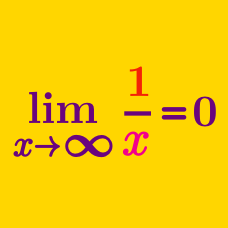Calculus

# Limits of Functions: Level 1 Challenges

$\boxed{\begin{array}{c|r:r:r:r:r} x & 0.1 & 0.01& 0.001& 0.0001& 0.00001\\ \hline \lfloor x \rfloor & 0 & 0 & 0 & 0 & 0 \end{array}}$

By looking at the table above, is it true that as $x$ approaches 0, then $\lfloor x \rfloor$ approaches 0 as well?
That is, is $\displaystyle \lim_{x\to0} \lfloor x\rfloor = 0$ correct?

 Notation: $\lfloor \cdot \rfloor$ denotes the floor function.

$\Large \lim_{x\to0} \dfrac{x}{x!} = \, ?$

Note: Treat $x! = \Gamma(x+1)$.

$\lim_{x\to\infty} \dfrac{\sin x}x = \, ?$

Find $\displaystyle\lim_{x\rightarrow 3}$ $\dfrac{x^2-9}{x-3}$

$\large \lim_{x \to 1} \dfrac{|x-1|}{x-1} = \, ?$

×